# Questions on rotation for JEE Main/Advanced and AP physics

In this page we have Questions on rotation for JEE Main/Advanced and AP physics . Hope you like them and do not forget to like , social share and comment at the end of the page.
A Rod of length L and Mass M can freely rotate around a pivotal A. A bullet of mass m and velocity v hit the rod at a height h from A and becomes embedded in it
Question 1 Find the angular velocity of the Rod just after the collision
a)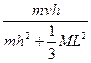b)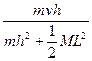c)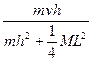d)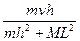Question 2 Find the Linear momentum of the system just after the collision if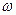is the angular velocity
a)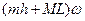b)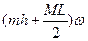c)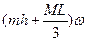d)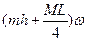An Upright hoop is projected on a pavement with a initial horizontal speed v0 but without spin so that its slides. The resulting frictional force causes the hoop to spin to loose translational speed and to acquire an angular speed. Eventually the hoop rolls without slipping
Let μ be the coefficient of friction.
Let M be the mass of the Upright hoop
Question 3 Find the time taken by the hoop to start rolling without slipping
a)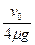b)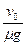c)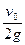d)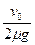Question 4 Find the velocity of the hoop at no slipping
a)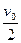b)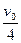c)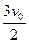d)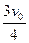Question 5 which one of the following statement are correct
a)  The loop will have total energy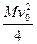when it start pure rolling
b)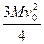Energy is lost in friction during the whole motion
c) The velocity of the top most point is v0 when it start pure rolling
d)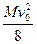is the rotational energy of the loop when it start pure rolling
Multiple Choice Questions
Question 6:
A nearly massless rod is pivoted at one end so it can freely swing as a pendulum. Two masses 2m and m are attached to it at distance a and 3a respectively from the pivot end. The rod is held horizontal and then released.
Which of the following is correct?
a) The angular acceleration at the instant it is released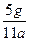b) Moment of inertia of the system about pivot is 11ma2
c) The angular acceleration at the instant when the rod makes an angle θ with horizontal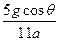d)  The net torque about the pivot end becomes zero when rod becomes vertical.
A cylinder of Mass M and radius R is kept on the plank. The plank starts moving with acceleration a. The cylinder rolls without slipping on the plank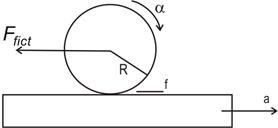Question 7 Find the acceleration of the cylinder center of mass from the laboratory frame of reference
a)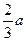b)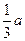c)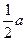d)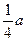Question 8 Find the frictional force acting on the cylinder
a)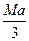b)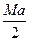c)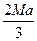d)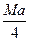Question 9 Find the angular acceleration of the cylinder about the axis passing through center of mass
a)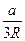b)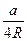c)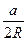d)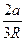Multiple Choice Questions
Question 10:
Two masses m1 and m2 separated by thin rod of length L. The system is shown below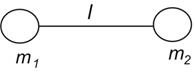The rod mass can be neglected
The system is thrown into the air. It is assumed only gravitational forces are acting and frictional forces are assumed to be null
Which of the following statement is true?
a) The center of mass of the system will follow a parabolic path
b)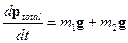c) The acceleration of the center of mass will be vertically downward and equal to g
d) None of these

Solutions